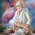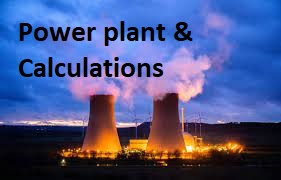### Calculation of heat rate & efficiency of the power plant

Heat rate is the amount of energy used by an electrical generator/power plant to generate one kilo Watt-hour (kWh) of electricity
Heat rate (HR) = Heat input / Power generation =Kcal / Kwh

Total heat input:
The chemical energy available in the fuel (coal, biomass, oil, gas etc) is converted into heat energy in Boilers, this process is called as oxidation. The heat available in the fuel is measured in terms of Kcal/kg, KJ/kg or BTU units. The part of this fuel is used as useful heat and rest is lost as dry flue gas loss, moisture loss, un  burnt loss, radiation/convection losses etc.Based on Boiler efficiency this heat energy from the fuel is utilised, generally fuel heat utilisation is in the range of 60 to 90%.
This heat generated in the boilers due to oxidation of fuel is used to generate high pressure & temperature steam. Thus generated steam is fed into the steam Turbine, where this heat energy also called thermal energy gets converted into Kinetic energy then into Mechanical energy in steam turbine, finally mechanical energy into electrical energy in Generator.
So total heat input to power plant =Chemical energy + Thermal energy + Kinetic energy+Mechanical energy
Output =Electrical power in Kwh
Heat rate =Heat input / Power generation
Efficiency:
Efficiency is nothing but the ratio of the useful work done to the heat provided. This means the friction and other losses are subtracted from the work done by thermodynamic cycles.

In Boilers efficiency = Heat out from the Boiler/Heat input to the Boiler
Heat output is Thermal energy in steam and heat in put is calorific value present in the fuel
In case of Turbine, Efficiency =860 X 100/Turbine heat rate

Case-1: Gross heat rate of Thermal power plant
In thermal power plants all the thermal energy generated from the steam generators/Boilers is used for only power generation.
Example: A 100 MW thermal power plant is running on 100% PLF, which consumes around 55 MT of coal having GCV 4500 kcal/kg per hour, then calculate the Gross station heat rate of the plant
We have,
Gross station heat rate =Heat input to the plant / Power generation

=Fuel consumed (MT)X GCV (kcal/kg)of fuel/Power generation/MWH

= (55 X 4500)/100

= 2475 kcal/kwh
Above problem can be solved by converting fuel consumed in kg/hr & power generation in
Kwh, then heat rate can be calculated as,
=55 X 1000 X 4500/(100 X 1000) =2475 kcal/kwh

Case-II
Station heat rate of Co-generation plant
In cogeneration plant thermal energy is used for process requirement and power generation. In cogeneration plant there are various sources of heat input & output to the station & from the station, where as in Thermal power plants heat input & output sources are only one.
Heat input to the station is in the form of heat energy present in fuel, make up water and return condensate from the process.
Heat output from the station is in the form of heat energy in process steam & power generation
Cogeneration heat rate = (Fuel consumed (MT) X GCV of fuel (kcal/kg + Quantity of return condensate from process (MT) X its enthalpy (kcal/kg) + Quantity of makeup water (MT) x its enthalpy kcal/kg)-(Process steam quantity (MT) X its enthalpy in kcal/kg) /Power generation in MW

Example: A process based cogeneration plant has following data on full day case study. Calculate the station heat rate
 Sl No. Particular Value 1 Power generation 977 MW 2 Total coal consumption Q1 875 MT 3 Gross calorific value of coal G 5100 kcal/kg 4 Steam given to process-1 plant at 2 kg/cm2g and 135 0C Q2 3720 MT 5 Steam given to process-2 plant at 7 kg/cm2g and 175 0C Q3 192 MT 6 Return condensate from process-1 plant at temperature 120 0C Q4 3350 MT 7 Return condensate from process-2 plant at temperature 85 0C Q5 135 MT 8 DM water make up to boiler at temperature 25 0C Q6 490 MT

From above given data we have,
Enthalpy of steam given to process plant-1 h2= 666.71 kcal/kg……..Refer steam table
Enthalpy of steam given to process plant-2 h3= 651.68kcal/kg
Enthalpy of process-1 return condensate h4 = 120.3 kcal/kg
Enthalpy of process-2 return condensate h5 = 85 kcal/kg
Enthalpy of makeup water h6 = 25 kcal/kg
We have station heat rate = ((Fuel consumption X GCV +Heat content in return condensate + Heat content in makeup water-Sum of heat content in process steam))/Power generation.
= (( Q1X G + Q4 X h4+Q5X h5 + Q6X h6)-(Q2 X h2+Q3 X h3))/Power generation
= ((875 X 5100+3350 x 120.3+135 x 85 +490 x 25)-(3720 x 666.71+192 x 651.68))/977
= 2337.71 kcal/kg

Turbine heat rate and efficiency:

Case-I: Turbine heat rate of a thermal power plant during performance guarantee (PG) test
Turbine Heat Rate (THR) = Steam flow X (Enthalpy of steam-Enthalpy of feed water)/Power generation
Case-II: Turbine heat rate of a thermal power plant during normal O&M condition
Turbine Heat Rate (THR) = (Steam flow X Enthalpy of steam-Feed water flow X Enthalpy of feed water)/Power generation
Turbine efficiency is given by
Turbine efficiency =860 X 100/Turbine heat rate
Example: A 22 MW Turbine has inlet steam flow 100 TPH at pressure & temperature 110 kg/cm2 & 535 Deg C respectively, then calculate the Turbine heat rate in both PG test case & O&M condition, also calculate Turbine efficiency in both the cases. Consider feed water temperature at economiser inlet is 195 deg c & flow is 102 TPH.
Solution:
Turbine inlet steam enthalpy at operating pressure & temperature H1 =824 kcal/kg
Feed water enthalpy =H2=198.15 kcal/kg
Steam flow =100TPH
Power generation =22 MW
Turbine heat rate of a thermal power plant during performance guarantee (PG) test
Turbine Heat Rate (THR) =(100 X (824-198.15)/22) =2844.77 kcal/kwh
Turbine efficiency =(860 X 100)/2844.77 =30.23%
Turbine heat rate of a thermal power plant during normal O&M condition
Turbine Heat Rate (THR) =(100 X 824-102 X 198.15)/22 =2826.25 kcal/kg
Turbine efficiency =(860 X 100)/2826.25 =30.42%
Case-III: Cogeneration Turbine heat rate
In case of Co-gen, Turbine heat rate is calculated by considering extractions and return condensate received.
Formula-1
Co-gen-THR =((Turbine inlet steam flow X its Enthalpy)-(Process steam flow X Enthalpy Exhaust steam flow X Enthalpy))Power generation
Formula-2
Co-gen-THR =((Turbine inlet steam flow X its Enthalpy + Process return condensate flow X its Enthalpy + Make up water flow X Its enthalpy)-(Process steam flow X Enthalpy + Feed water flow X Enthalpy))Power generation
Example:21 MW condensing cum extraction turbine has inlet steam flow 120 TPH at 88 kg/cm2g pressure and 520 0C temperature, it has two extraction first, at 16 kg/cm2g pressure and temperature 280 0C at flow 25 TPH and second at 2.5 kg/cm2g pressure and temperature 150 0C at flow 75 TPH.Remaining steam goes to condenser at exhaust pressure 0.09 kg/cm2a.Calculate the turbine heat rate and thermal efficiency by using both formulae. Consider steam given to process is 10 TPH less than each extraction, the return condensate from the process is 70 TPH at temperature 90 deg c, feed water flow 122 TPH at temperature 195 deg c & make up water flow 13 TPH at temp 28 deg c.
Given that,
Power generating capacity of turbine = 21 MWH
Q1 = 120 TPH
Enthalpy h1 at 88 kg/cm2g and 5200C = 820.66 kcal/kg
Q2 = 25 TPH
h2 at 16 kg/cm2g and 2800C  = 715.88 kcal/kg
Q3 = 75 TPH
h3 at 2.5 kg/cm2g and 1500C  = 658.40 kcal/kg
Condenser steam flow Q4 = Q1-Q2-Q3 = 120-25-75 = 20 TPH
h4 at exhaust pressure =  44.06 kcal/kg
Formula-1
Cogen-Turbine heat rate (THR) = (Input heat to turbine- Sum of extraction and exhaust heat)/Power generation
= ((Q1 X h1)-(Q2 X h2+Q3 X h3 +Q4 X h4))/Power generation

= ((120 X 820.66)-(25 X 715.88 +75 X 658.40 + 20 X 44.06))/21

= 1443.85 kcal/kwh

Turbine thermal efficiency = (860 X 100)/Turbine heat rate

= (860 x100)/1443.85

= 59.56%
Formula-2

Co-gen-THR =((Turbine inlet steam flow X its Enthalpy + Process return condensate flow X its Enthalpy + Make up water flow X Its enthalpy)-(Process steam flow X Enthalpy + Feed water flow X Enthalpy))Power generation
THR =((120 X 820.66 +90 X 90 +13 x 28)-(15 X 715.88 +65 X 658.40 + 120 X 198.15))/21
THR =1495.73 kcal/kwh
Turbine thermal efficiency = (860 X 100)/Turbine heat rate

= (860 x100)/1495.73

= 57.49%

1.In the example ,heat rate during PG test is higher than normal O&M condition with increased feed water flow of 102 t. how it can be possible? In my calculation the heat rate will become 2882.52 kcal/kwh from 2844kcal/kwh and turbine efficiency will also decreased from 30.23% to 29.83%.

1.Feed water flow will be always more than steam generation..Considered 2 TPH i.e 2% as blow down loss

2.Sir according to my experience mean of feed flow is less than the mean of steam flow

3.In such case, attemperator water flow might have calculated separately.

In all cases, steam generated = Feed water flow + Attemperator water flow

2.By calculating plant heat rate indirect method, I am getting 2691kcal/kwh. Where as direct method I am getting 2760 kcal/kwh. Why I am getting this difference and what are the reasons.

3.Very very useful

4.Nice to be visiting your blog again, it has been months for me. Well this article that i've been waited for so long. I need this article to complete my assignment in the college, and it has same topic with your article. Thanks, great share. carbon monoxide

Hi all,